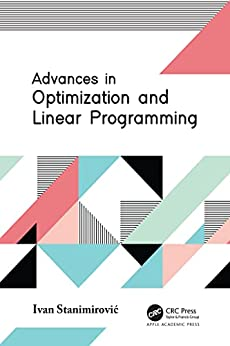Advances in Optimization and Linear ProgrammingEnglish | 2022 | ISBN: ‎ 1774637405 | 204 pages | True PDF | 1.99 MB

This new volume provides the information needed to understand the simplex method, the revised simplex method, dual simplex method, and more for solving linear programming problems.
Following a logical order, the book first gives a mathematical model of the linear problem programming and describes the usual assumptions under which the problem is solved. It gives a brief description of classic algorithms for solving linear programming problems as well as some theoretical results. It goes on to explain the definitions and solutions of linear programming problems, outlining the simplest geometric methods and showing how they can be implemented. Practical examples are included along the way. The book concludes with a discussion of multi-criteria decision-making methods.
Advances in Optimization and Linear Programming
is a highly useful guide to linear programming for professors and students in optimization and linear programming.